# Analysis of Impact Behaviour of Sisal-Epoxy Composites under Low Velocity Regime

Analysis of Impact Behaviour of Sisal-Epoxy Composites under Low Velocity Regime

Vishwas MaheshAshutosh Nilabh Sharnappa Joladarashi Satyabodh M. Kulkarni

Department of Industrial Engineering and Management, Siddaganga Institute of Technology, Tumakuru 572103, India

Department of Mechanical Engineering, National Institute of Technology Karnataka, Surathkal, Mangaluru 575025, India

Corresponding Author Email:
vishwasm@sit.ac.in
Page:
57-63
|
DOI:
https://doi.org/10.18280/rcma.310108
13 August 2020
|
Revised:
24 December 2020
|
Accepted:
7 January 2021
|
Available online:
28 February 2021
| Citation

OPEN ACCESS

Abstract:

The present study concentrates on development of conceptual proof for sisal reinforced polymer matrix composite for structural applications subjected to low velocity impact using a finite element (FE) approach. The proposed sisal-epoxy composite of various thicknesses of 3.2 mm, 4 mm and 4.8 mm is subjected to different impact velocities of 1 m/s, 2 m/s and 3 m/s ranging in the low velocity impact regime to study the energy absorbed and damage mitigation behaviour of the proposed composite. The consequence of velocity of impact and thickness of laminate on the sisal epoxy composite’s impact behaviour is assessed statistically using Taguchi’s experimental design. Outcome of the present study discloses that the energy absorption increases with increased impact velocity and laminate thickness. However, the statistical study shows that impact velocity is predominant factor affecting the impact response of sisal epoxy composite laminate compared to laminate thickness. The role of matrix and fiber in damage initiation is studied using Hashin criteria and it is found that matrix failure is predominant over the fiber failure.

Keywords:

impact, sisal-epoxy composite, damage mitigation, finite element, Taguchi, DOE

1. Introduction

In recent years the use of polymer based composite materials has significantly increased due to advantage in its properties such as specific stiffness and specific strength. They also possess better environmental and corrosion resistance which is an added advantage. All these factors make the polymer matrix composites a potential replacement material for conventional materials. Structural components making use of composites needs to be monitored regularly since they suffer impact loading leading to serious damage . The impact behaviour of the composites depends on various factors such as thickness of the laminate, orientation of the fibers, weight of the impactor, shape of the impactor and velocity of the impactor [2, 3].

The laminate’s thickness and shape of the impactor effect on the impact behaviour of jute-epoxy composite was assessed using finite element method by Mahesh et al.  and was found that laminate thickness played a major role in deciding the energy absorption of the composite. It was also found that the damage area in the composite is more when impacted by flat impactor followed by hemispherical and conical impactor.

The effect of the shape of the impactor on the impact response of sandwich composite was studied by Liu et al.  using finite element approach. It was found that the sandwich composite when impacted with conical impactor absorbs more energy compared to flat and hemispherical.

The appropriateness of jute epoxy composite laminate for low and ballistic velocity impacts was studied by Mahesh et al.  and was found that jute-epoxy composite is better suitable for low velocity impact rather than ballistic impact. The extent to which the damage resistance of the composite is enhanced mainly depends on the impact velocity . Thus, it is essential to assess the role of impact velocity on the impact behaviour of the composite.

Natural fibers are finding a prominent place as reinforcements in polymer matrix composites replacing the synthetic fibers due to their better properties such as biodegradability, low cost, ease of availability and acceptable mechanical properties as opposed to synthetic fibers [7–11]. Top automobile giants like Mercedes Benz is using natural fiber jute based composite for their door panels just because of its light weight and low cost. Various natural fibers are available to be used in composites, out of which jute, coir and bamboo are commonly used for structural applications in automobiles. Though sisal has similar properties which are comparable with jute, its use in structural applications is limited. Sisal fiber exhibits low density and when reinforced with epoxy composite exhibits the higher specific strength which is comparable with glass epoxy composite .

Sisal is a natural fiber consisting of cellulose (78% wt), lignin (8%), hemicelluloses (10%), waxes (2%) and ash (1%). The sisal is a hard fiber whose length varies between 1 to 1.5 m and thickness between 0.1 to 0.3 mm . Epoxy is a thermoset based matrix exhibiting better adhesion and hence used as structural adhesives for engineering applications. They exhibit better resistance to heat and chemical reactions.

Suitability of the material must be checked before finalizing it for any applications. Usage of experimental methods is costly during initial stage of selection process, which consumes lot of time. Top overcome this disadvantage, engineers and designers prefer to use finite element approach [5, 14-20]. The aim of this study is therefore to develop the conceptual proof and to determine the impact behaviour of sisal-epoxy composite subjected to low velocity impact. Also, the effect of impact velocity and laminate thickness on the energy absorption and damage mitigation behaviour of the proposed composite is studied.

2. Modelling and Simulation

This section deals with details pertaining to modelling and simulation adopted in the present study. The low velocity impact response of the proposed sisal-epoxy composite is carried out using commercially available FE software. Shell type element SC8R is used to model the sisal-epoxy laminate as deformable body with four layers and each layer having thickness of 8 mm. The surface to surface contact is defined for each layers of the laminate. The laminates are modelled with a dimension of 125 mm x 75 mm as per ASTM D7136 standard. The impactor is modelled as rigid body using R3D4 element type. The pictorial representation pertaining to modelling and meshing of the laminate and impactor is presented through Figure 1.

Pertaining to the boundary condition, the laminate is constrained in all six degrees of freedom on its edges and the impactor is assigned a mass of 1 kilogram with movement allowed only in downward direction. Impact velocity of 1m/s, 2m/s and 3m/s is assigned to the impactor. The boundary conditions assigned are shown in Figure 2.

Integrity between the laminate and impactor is maintained using contact penalty contact algorithm with hard contact, coefficient of friction 0.3 and general contact algorithm was made use of to define the contact between the layers as used in the literature . The material properties used for sisal-epoxy composite used in FE analysis is taken from the literature [13, 22, 23] and tabulated in Table 1. The adopted methodology has been validated for the results of impact response of jute epoxy composites carried out by Mahesh et al. .

## 1.png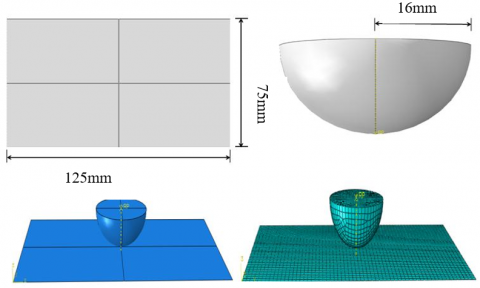Figure 1. Modelling, assembly and meshing of impactor and laminate

## 2.jpgFigure 2. Boundary conditions assigned to laminate and impactor

Table 1. Sisal-Epoxy material properties used in FE analysis [13, 22, 23]

 Density N12 E1 E2 G12=G31=G23 XT XC YT YC (Kg/m3) (MPa) 1310 0.27 456 180 208 102 11.5 1.3 20.1

The damage of the proposed sisal epoxy composite is governed by Hashin’s criteria .

In order to assess the effect of laminate thickness and impact velocity on the impact response of the proposed sisal epoxy composite laminate, Taguchi’s Design of Experiments (DOE) with L9 orthogonal array is made use. The factors and their levels used for DOE is presented in Table 2.

Table 2. Factors and levels used in Taguchi’s DOE

 Factors Level 1 Level 2 Level 3 Impact Velocity (m/s) 1 2 3 Laminate Thickness (mm) 3.2 4 4.8
3. Results and Discussions

The obtained results are made use of to assess the energy absorption of the laminate, maximum force the laminate can withstand and the nature of damage the laminate has undergone at different impact velocities.

3.1 Energy absorption

The proposed sisal-epoxy composite is subjected to low velocity impact at 1m/s, 2m/s and 3m/s resulting in impact kinetic energy of 0.5 J, 2J and 4.5J. The impact energy at which the impactor strikes the laminate is given by Eq. (1).

$E_{K E}=\frac{1}{2} m v^{2}$     (1)

where, EKE is kinetic energy, m is impactor mass in Kgs and v is the velocity of impact in m/s.

Once the impactor strikes the laminate, the impactor’s kinetic energy dissipates in the laminate in the form of various types of damage. This further reduces the velocity of the impactor. The impactor can further rebound or penetrate from the laminate at certain velocity termed as residual velocity vr with residual energy Er. The energy absorbed by the laminate is provided by Eq. (2) and residual energy is calculated using Eq. (3).

$E_{a}=E_{K E}-E_{r}$     (2)

$E_{r}=\frac{1}{2} m v_{r}^{2}$     (3)

The energy absorption of the proposed sisal epoxy composite of different thicknesses are presented in Table 3.

Table 3. Energy absorbed by sisal-epoxy laminate at different impact velocities

 Impact velocity (m/s) Impact energy (J) Thickness (mm) Residual velocity (m/s) Residual energy (J) Energy absorbed (J) 1 0.5 3.2 0.67 0.23 0.27 2 2 1.28 0.82 1.18 3 4.5 1.34 1.9 2.60 1 0.5 4 0.54 0.15 0.35 2 2 1.04 0.54 1.46 3 4.5 1.82 1.66 2.84 1 0.5 4.8 0.28 0.04 0.46 2 2 0.64 0.21 1.79 3 4.5 1.04 0.55 3.95

Table 4. Peak force of the sisal-epoxy composite at different impact velocity

 Impact velocity (m/s) Impact energy (J) Thickness (mm) Peak force (N) 1 0.5 3.2 200 2 2 390 3 4.5 670 1 0.5 4 211 2 2 398 3 4.5 683 1 0.5 4.8 219 2 2 409 3 4.5 697

The variation of energy absorption capability of sisal epoxy composite with varied thickness for the purpose of comparison is presented in Figure 3.

## 3.png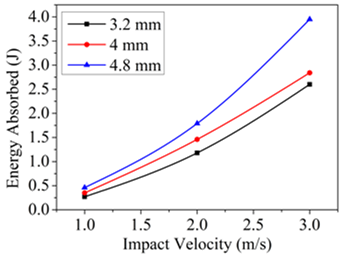Figure 3. Variation in energy absorption of sisal epoxy composite laminate of different thickness

It can be analysed from the Figure 3 that as laminate thickness increases, the energy absorption capability of the sisal-epoxy composite is increased. At a velocity of impact of 3 m/s, it can be seen that energy absorption of laminate with 4.8 mm thickness is much better than its counterparts. The laminate with 4.8 mm thickness absorbs nearly 52% and 39% more energy compared to laminates with thickness of 3.2 mm and 4 mm respectively. This is due to the fact that at higher impact velocity, the extent of damage that the laminate undergoes is higher leading to maximum energy absorption.

3.2 Force response

Peak force is obtained from the force time response curve which is an indication of the ability of the composite to resist damage and represents the load bearing capability of the composite. The peak force obtained for sisal-epoxy composite laminate at different impact velocities and different thickness is tabulated in Table 4.

## 4.png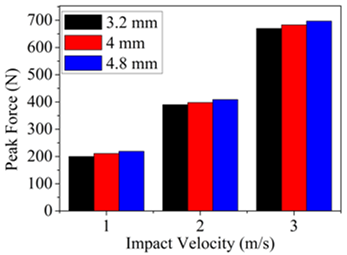Figure 4. Variation in peak force of sisal epoxy composite laminate of different thickness

Figure 4 shows the variation in peak force of sisal epoxy composite laminate of different thickness. It can be seen from the obtained results that with increase in thickness of the laminate, the peak force increases indicating that the composite is capable of withstanding the maximum load or is capable of resisting the damage at higher thickness. The peak force for the laminate of thickness 4.8 mm at an impact velocity of 3 m/s is nearly 4% and 2% higher than laminates of thickness 3.2 mm and 4 mm respectively. The continuous increase in the peak force of the composite laminate is an indication that the saturation in peak force is yet to take place [24, 25]. This is an indication that the proposed flexible composite can still withstand higher impact energy.

3.3 Taguchi approach

Taguchi’s DOE approach is adopted to study the influence of the thickness and impact velocity on the energy absorption and peak force behaviour of the proposed sisal epoxy composite. The proposed sisal epoxy composite laminate is expected to exhibit better energy absorption and higher peak force. Thus, SN ratio with larger the better criteria according to Eq. (4) is used.

$S N=-10 \log \left(\Sigma\left(1 / y^{2}\right) / n\right)$    (4)

where, ‘y’ is the responses for the given factor level combination and ‘n’ is the number of responses in the factor level combination.

Figure 5 shows the mean effect plots for SN ratio for energy absorbed and force form which it is clear that for better energy absorption and better damage resistance, the thickness of the laminate and impact velocity should be high (level 3). This means that the combination of sisal epoxy composite laminate with 4.8 mm thickness when impacted with 3 m/s provides better response.

The response table for SN ratios is shown in Table 5. From the response table of SN ratio, it is evident that among the impact velocity and laminate thickness, impact velocity is the factor that affects the response of the sisal epoxy composite laminate compared to thickness. Thus is supported by ANOVA as tabulated in Table 6. It can be seen that the percentage contribution of impact velocity for both energy absorption and force is 90.36% and 99.78% respectively.

The regression equation obtained statistically for energy absorbed and force is presented through Eq. (5) and Eq. (6) respectively.

Energy Absorbed $=-1.831+1.385$ Impact Velocity + 0.358 Laminate Thickness     (5)

Force $=-64.2+236.7$ Impact Velocity +10.8 Laminate Thickness     (6)

## 5-1.png## 5-2.pngFigure 5. Mean effect plots for SN ratio for (a) Energy absorbed and (b) Force

Table 5. SN ratio for various factors at different levels

 Energy Absorbed Force Level SN Ratio for Impact Velocity SN Ratio for Laminate Thickness SN Ratio for Impact Velocity SN Ratio for Laminate Thickness 1 -9.0787 -0.5452 46.44 51.45 2 3.2606 1.0783 52.02 51.72 3 9.7659 3.4147 56.69 51.97 Delta 18.8447 3.9599 10.25 0.51 Rank 1 2 1 2

Table 6. ANOVA for energy absorbed and force

 Source Response DF SS MS F value P Value % Contribution Impact Velocity Energy Absorbed 2 11.6534 5.8267 55.29 0.001 90.36 Force 2 340611 170305 27868.16 0.000 99.78 Laminate Thickness Energy Absorbed 2 0.8206 0.4103 3.89 0.115 0.00063 Force 2 704 352 57.62 0.001 0.2062 Error Energy Absorbed 4 0.4215 0.1054 0.00032 Force 4 24 6 7.031x10-7 Total Energy Absorbed 8 12.8954 100 Force 8 341340 100 Energy Absorbed : S = 0.454985, R-Sq = 90.37%, R-Sq (Adj) = 87.16%, R-Sq (Pred) =78.33% Force: S = 2.47207, R-Sq = 99.99%, R-Sq (Adj) = 99.99%, R-Sq (Pred) =99.96%

3.4 Damage study

Since it was found from the Taguchi analysis that impact velocity is the predominant factor affecting the impact response of the proposed sisal epoxy composite, the extent of damage caused in the sisal-epoxy composite laminate is assessed at different impact velocities in terms of Hashin failure criteria and is presented in Figure 6.

Hashin failure criteria distinguish among the various different failure modes of the unidirectional lamina: tensile and compressive fiber failures and tensile and compressive matrix failure, and they are given separately for each of these failure modes. Hashin failure criteria is made use of to anticipate the failure in the sisal-epoxy composite laminate used in the present study. The different failure modes considered in the present study are produced using Eq. (7-10).

\begin{aligned} &\text { Fiber Tension }\\ &\left(\hat{\sigma_{11}} \geq 0\right) \quad F_{f}^{t}=\left(\frac{\hat{\sigma_{11}}}{X^{t}}\right)^{2}+\alpha\left(\frac{\hat{\sigma_{12}}}{S^{L}}\right)^{2} \end{aligned}     (7)

\begin{aligned} &\text { Fiber Compression }\\ &\left(\hat{\sigma_{11}} \leq 0\right) \quad F_{f}^{c}=\left(\frac{\hat{\sigma_{11}}}{X^{c}}\right)^{2} \end{aligned}     (8)

\begin{aligned} &\text { Matrix Tension }\\ &\left(\hat{\sigma_{22}} \geq 0\right) \quad F_{m}^{t}=\left(\frac{\hat\sigma_{22}}{Y^{t}}\right)^{2}+\left(\frac{\hat\sigma_{12}}{S^{L}}\right)^{2} \end{aligned}     (9)

\begin{aligned} &\text { Matrix Compression }\\ &\left(\hat\sigma_{22} \leq 0\right) \quad F_{m}^{c}=\left(\frac{\hat\sigma_{22}}{2 S^{T}}\right)^{2}+\left[\left[\frac{Y^{c}}{2 S^{T}}\right]^{2}-1\right]\left(\frac{\hat\sigma_{22}}{Y^{c}}\right)+\left(\frac{\hat\sigma_{12}}{S^{L}}\right)^{2} \end{aligned}     (10)

In Eq. (7-10), $\hat\sigma_{i j}$ (i, j = 1, 2) represents the effective stress tensor components. The tensile and compressive strength of the laminate are represented by Xt, Xc in longitudinal direction and Yt, Yc in transverse direction. The in plane and out of plane shear strength of the laminate are represented by Sj (j = L,T). The material, before initiation of damage will behave linear elastic during which the stress-strain can be related as $\{\sigma\}=[C]\{\varepsilon\}$ where [C] is the elasticity matrix which changes to damage elasticity matrix [Cd] once the damage is initiated. The damage elasticity matrix is defined as in Eq. (11) below

$\left[C_{d}\right]=\left[\begin{array}{ccc} \left(1-d_{f}\right) E_{1} & \left(1-d_{f}\right)\left(1-d_{m}\right) \gamma_{21} E_{1} & 0 \\ \left(1-d_{f}\right)\left(1-d_{m}\right) \gamma_{12} E_{2} & \left(1-d_{m}\right) E_{2} & 0 \\ 0 & 0 & \left(1-d_{s}\right) G_{12} D \end{array}\right]$     (11)

where, $D=1-\left(1-d_{f}\right)\left(1-d_{m}\right) \gamma_{12} \gamma_{21}$ and $d_{f}, d_{m} \text { and } d_{s}$  are current states of fiber damage, matrix damage and shear damage respectively.

Analysing the damage plots, it is evident that with increase in the impact energy, the extent of damage increases in all the four different modes of damage considered by Hashin criteria. Due to low velocity impact, the compression of the fibers is initiated at the point of contact of the impactor on the laminate and the fiber beneath the impactor experience the compressive force which propagates outwards as the impact energy is increased. However, the fibers away from the impactor which are at the boundaries of the smaller side of the laminate experience the tensile forces and the tensile stresses propagate inwards towards the point of impact as the impact energy is increased. The fiber failure occurs either due to fiber pull out or fiber breakage during an impact event. In case of matrix, during an impact event, the matrix surrounding the impactor experiences the compressive stresses and the delamination stats to initiate in ‘X’ shaped manner. Increase in the impact energy results in stresses being propagating towards the outer edge of the “X” shaped damage leading to complete delamination at the point away from the impact. On the other hand, the matrix also undergoes tension with delamination and cracking creating away from the point of impact and further propagating towards the point of impact as the impact energy is increased. It is evident that matrix failure is predominant over the fiber failure in case of sisal-epoxy composite subjected to low velocity impact loading.

## 6-1.png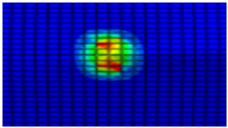Figure 6(a). Fiber compression at impact velocity of 1 m/s

## 6-2.png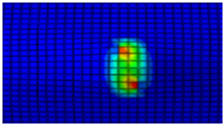Figure 6(b). Fiber compression at impact velocity of 2 m/s

## 6-3.png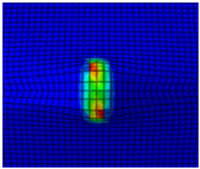Figure 6(c). Fiber compression at impact velocity of 3 m/s

## 6-4.png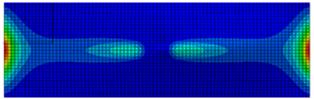Figure 6(d). Fiber tension at impact velocity of 1 m/s

## 6-5.png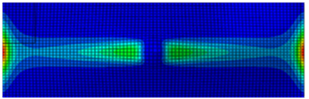Figure 6(e). Fiber tension at impact velocity of 2 m/s

## 6-6.png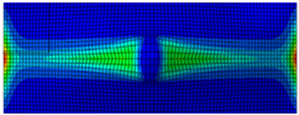Figure 6(f). Fiber tension at impact velocity of 3 m/s

## 6-7.png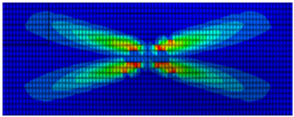Figure 6(g). Matrix compression at impact velocity of 1 m/s

## 6-8.png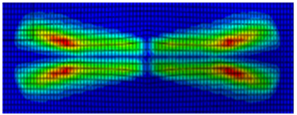Figure 6(h). Matrix compression at impact velocity of 2 m/s

## 6-9.png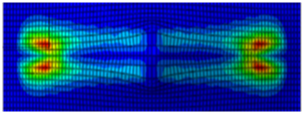Figure 6(i). Matrix compression at impact velocity of 3 m/s

## 6-10.png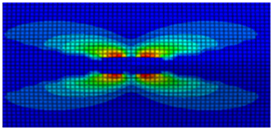Figure 6(j). Matrix tension at impact velocity of 1 m/s

## 6-11.png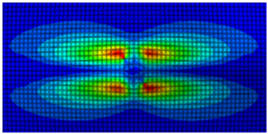Figure 6(k). Matrix tension at impact velocity of 2 m/s

## 6-12.pngFigure 6(l). Matrix tension at impact velocity of 3 m/s

Figure 6. Hashin damage initiation plot for sisal epoxy composite laminates

4. Conclusions

The FE and statistical study of the sisal-epoxy composite subjected to low velocity impact at different impact velocities has been carried out in order to propose a conceptual proof of the sisal-epoxy composite for structural applications. The impact response of the proposed composite is analysed and following conclusions are drawn:

• It is found that energy absorption of laminate with 4.8 mm thickness is much better than its counterparts. The laminate with 4.8 mm thickness absorbs nearly 52% and 39% more energy compared to laminates with thickness of 3.2 mm and 4 mm respectively.
• The peak force of the composite laminate increases with increase in thickness of the laminate. The peak force for the laminate of thickness 4.8 mm at an impact velocity of 3 m/s is nearly 4% and 2% higher than laminates of thickness 3.2 mm and 4 mm respectively. The proposed flexible composite can still take more load as the peak force with varied impact velocities is not saturated.
• Taguchi’s DOE shows that impact velocity is the predominant factor that affects the impact response of the laminate compared to laminate thickness.
• Analysing the Hashin damage initiation plots, it is evident that compression of the fibers is initiated at the point of contact of the impactor on the laminate and compressive force propagates outwards as the impact energy is increased. Fibers away from the impact zone experience the tensile stresses. The fibers fail either due to fiber breakage or fiber pullout.
• In case of matrix, the failure of the matrix takes place due to matrix cracking leading to delamination. It is evident that matrix failure is predominant over the fiber failure in case of sisal-epoxy composite subjected to low velocity impact loading.
Acknowledgement

The author acknowledges the support from the Department of Science and Technology (DST) file no. ECR/2016/001448 funded by Science and Engineering Research Board, Government of India and also acknowledges the support and facilities provided by Smart Structure Laboratory, National Institute of Technology Karnataka, India.

References

 Mahesh, V., Joladarashi, S., Kulkarni, S.M. (2020). Influence of laminate thickness and impactor shape on low velocity impact response of jute-epoxy composite: FE study. Materials Today: Proceedings, 28 (Part 2): 545-550. https://doi.org/10.1016/j.matpr.2019.12.216

 Caminero, M.A., Rodríguez, G.P. (2018). Experimental study of the influence of thickness and ply-stacking sequence on the compression after impact strength of carbon fibre reinforced epoxy laminates. Polymer Testing, 66: 360-370. https://doi.org/10.1016/j.polymertesting.2018.02.009

 Katz, S., Grossman, E., Gouzman, I., Murat, M., Wiesel, E., Wagner, H.D. (2008). Response of composite materials to hypervelocity impact. International Journal of Impact Engineering, 35(12): 1606-1611. https://doi.org/10.1016/j.ijimpeng.2008.07.032

 Liu, J.X., He, W.T., Xie, D., Tao, B. (2017). The effect of impactor shape on the low-velocity impact behavior of hybrid corrugated core sandwich structures. Composites Part B: Engineering, 111: 315-331. https://doi.org/10.1016/j.compositesb.2016.11.060

 Mahesh, V., Joladarashi, S., Kulkarni, S.M. (2018). Suitability study of jute-epoxy composite laminate for low and high velocity impact applications. AIP Conference Proceedings, 1943: 020106. https://doi.org/10.1063/1.5029682

 Ali, M., Joshi, S.C., Sultan, M.T.H. (2017). Palliatives for low velocity impact damage in composite laminates. Advances in Materials Science and Engineering, 2017: 1-16. https://doi.org/10.1155/2017/8761479

 Shah, D.U. (2014). Natural fibre composites: Comprehensive Ashby-type materials selection charts. Materials & Design (1980-2015), 62: 21-31. https://doi.org/10.1016/j.matdes.2014.05.002

 Dicker, M.P.M., Duckworth, P.F., Baker, A.B., Francois, G., Hazzard, M.K., Weaver, P.M. (2014). Green composites: A review of material attributes and complementary applications. Composites Part A: Applied Science and Manufacturing, 56: 280-289. https://doi.org/10.1016/j.compositesa.2013.10.014

 Faruk, O., Bledzki, A.K., Fink, H.P., Sain, M. (2012). Biocomposites reinforced with natural fibers: 2000-2010. Progress in Polymer Science, 37(11): 1552-1596. https://doi.org/10.1016/j.progpolymsci.2012.04.003

 Ahmad, F., Choi, H.S., Park, M.K. (2015). A review: Natural fiber composites selection in view of mechanical, light weight, and economic properties. Macromolecular Materials and Engineering, 300(1): 10-24. https://doi.org/10.1002/mame.201400089

 Mahesh, V., Joladarashi, S., Kulkarni, S.M. (2020). Damage mechanics and energy absorption capabilities of natural fiber reinforced elastomeric based bio composite for sacrificial structural applications. Defence Technology. https://doi.org/10.1016/j.dt.2020.02.013

 Sathishkumar, T.P., Navaneethakrishnan, P., Shankar, S., Rajasekar, R., Rajini, N. (2013). Characterization of natural fiber and composites - A review. Journal of Reinforced Plastics and Composites, 32(19): 1457-1476. https://doi.org/10.1177/0731684413495322

 Bisanda, E.T.N., Ansell, M.P. (1991). The effect of silane treatment on the mechanical and physical properties of sisal-epoxy composites. Composites Science and Technology, 41(2): 165-178. https://doi.org/10.1016/0266-3538(91)90026-L

 Ariatape, M.Y., Mashayekhi, M., Ziaei-Rad, S. (2014). Prediction of all-steel CNG cylinders fracture in impact by using ‎ damage mechanics approach. Scientia Iranica, 21(3): 609-619.

 Mahesh, V., Sharnappa, J., Kulkarni, S.M. (2019). Study on stacking sequence of plies in green sandwiches for low velocity impact application. Key Engineering Materials, 801: 59-64. https://doi.org/10.4028/www.scientific.net/KEM.801.59

 Mahesh, V., Joladarashi, S., Kulkarni, S.M. (2018). Finite element simulation of low velocity impact loading on a sandwich composite. MATEC Web Conf., 144: 1-10. https://doi.org/10.1051/matecconf/201714401010

 Mahesh, V., Joladarashi, S., Kulkarni, S.M. (2017). Modelling and analysis of material behaviour under normal and oblique low velocity impact. Materials Today: Proceedings, 5(2): 6635-6644. https://doi.org/10.1016/j.matpr.2017.11.319

 Mahesh, V., Joladarashi, S., Kulkarni, S.M. (2019). Comparative study on energy absorbing behavior of stiff and flexible composites under low velocity impact. AIP Conference Proceedings, 2057: 020025(1)-020025(6). https://doi.org/10.1063/1.5085596

 Mahesh, V., Joladarashi, S., Kulkarni, S.M. (2019). Investigation on effect of using rubber as core material in sandwich composite plate subjected to low velocity normal and oblique impact loading. Scientia Iranica, 26(2): 897-907. https://doi.org/10.24200/sci.2018.5538.1331

 Mahesh, V., Joladarashi, S., Kulkarni, S.M. (2020). Influence of thickness and projectile shape on penetration resistance of the compliant composite. Defence Technology. https://doi.org/10.1016/j.dt.2020.03.006

 Lopes, C.S., Camanho, P.P., Gürdal, Z., Maimí, P., González, E.V. (2009). Low-velocity impact damage on dispersed stacking sequence laminates. Part II : Numerical simulations. Composites Science and Technology, 69(7-8): 937-947. https://doi.org/10.1016/j.compscitech.2009.02.015

 Rong, M., Zhang, M., Liu, Y., Yang, G., Zeng, H. (2001). The effect of fiber treatment on the mechanical properties of unidirectional sisal- reinforced epoxy composites. Composites Science and Technology, 61(10): 1437-1447. http://dx.doi.org/10.1016/S0266-3538(01)00046-X

 Oksman, K., Wallström, L., Berglund, L.A., Toledo Filho, R.D. (2002). Morphology and mechanical properties of unidirectional sisal-epoxy composites. Journal of Applied Polymer Science, 84(13): 2358-2365. https://doi.org/10.1002/app.10475

 Kim, E.H., Rim, M.S., Lee, I., Hwang, T.K. (2013). Composite damage model based on continuum damage mechanics and low velocity impact analysis of composite plates. Composite Structures, 95: 123-134. https://doi.org/10.1016/j.compstruct.2012.07.002

 Mahesh, V., Joladarashi, S., Kulkarni, S.M. (2019). An experimental investigation on low-velocity impact response of novel jute/ rubber flexible bio-composite. Composite Structures, 225: 111190. https://doi.org/10.1016/j.compstruct.2019.111190